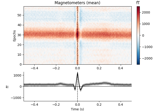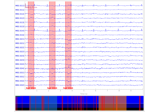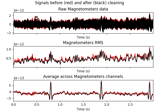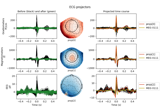# mne.preprocessing.create_ecg_epochs#

mne.preprocessing.create_ecg_epochs(raw, ch_name=None, event_id=999, picks=None, tmin=-0.5, tmax=0.5, l_freq=8, h_freq=16, reject=None, flat=None, baseline=None, preload=True, keep_ecg=False, reject_by_annotation=True, decim=1, verbose=None)[source]#

Conveniently generate epochs around ECG artifact events.

This function will:

1. Filter the ECG data channel.

2. Find ECG R wave peaks using `mne.preprocessing.find_ecg_events()`.

3. Create `Epochs` around the R wave peaks, capturing the heartbeats.

Note

Filtering is only applied to the ECG channel while finding events. The resulting `ecg_epochs` will have no filtering applied (i.e., have the same filter properties as the input `raw` instance).

Parameters:
rawinstance of `Raw`

The raw data.

ch_name

The name of the channel to use for ECG peak detection. If `None` (default), ECG channel is used if present. If `None` and no ECG channel is present, a synthetic ECG channel is created from the cross-channel average. This synthetic channel can only be created from MEG channels.

event_id`int`

The index to assign to found ECG events.

picks

Channels to include. Slices and lists of integers will be interpreted as channel indices. In lists, channel type strings (e.g., ```['meg', 'eeg']```) will pick channels of those types, channel name strings (e.g., `['MEG0111', 'MEG2623']` will pick the given channels. Can also be the string values “all” to pick all channels, or “data” to pick data channels. None (default) will pick all channels. Note that channels in `info['bads']` will be included if their names or indices are explicitly provided.

tmin`float`

Start time before event.

tmax`float`

End time after event.

l_freq`float`

Low pass frequency to apply to the ECG channel while finding events.

h_freq`float`

High pass frequency to apply to the ECG channel while finding events.

reject

Reject epochs based on maximum peak-to-peak signal amplitude (PTP), i.e. the absolute difference between the lowest and the highest signal value. In each individual epoch, the PTP is calculated for every channel. If the PTP of any one channel exceeds the rejection threshold, the respective epoch will be dropped.

The dictionary keys correspond to the different channel types; valid keys can be any channel type present in the object.

Example:

```reject = dict(grad=4000e-13,  # unit: T / m (gradiometers)
mag=4e-12,      # unit: T (magnetometers)
eeg=40e-6,      # unit: V (EEG channels)
eog=250e-6      # unit: V (EOG channels)
)
```

Note

Since rejection is based on a signal difference calculated for each channel separately, applying baseline correction does not affect the rejection procedure, as the difference will be preserved.

Note

To constrain the time period used for estimation of signal quality, pass the `reject_tmin` and `reject_tmax` parameters.

If `reject` is `None` (default), no rejection is performed.

flat

Reject epochs based on minimum peak-to-peak signal amplitude (PTP). Valid keys can be any channel type present in the object. The values are floats that set the minimum acceptable PTP. If the PTP is smaller than this threshold, the epoch will be dropped. If `None` then no rejection is performed based on flatness of the signal.

Note

To constrain the time period used for estimation of signal quality, pass the `reject_tmin` and `reject_tmax` parameters.

baseline`None` | `tuple` of length 2

The time interval to consider as “baseline” when applying baseline correction. If `None`, do not apply baseline correction. If a tuple `(a, b)`, the interval is between `a` and `b` (in seconds), including the endpoints. If `a` is `None`, the beginning of the data is used; and if `b` is `None`, it is set to the end of the interval. If `(None, None)`, the entire time interval is used.

Note

The baseline `(a, b)` includes both endpoints, i.e. all timepoints `t` such that `a <= t <= b`.

Correction is applied to each epoch and channel individually in the following way:

1. Calculate the mean signal of the baseline period.

2. Subtract this mean from the entire epoch.

preload`bool`

Preload epochs or not (default True). Must be True if keep_ecg is True.

keep_ecg`bool`

When ECG is synthetically created (after picking), should it be added to the epochs? Must be False when synthetic channel is not used. Defaults to False.

reject_by_annotation`bool`

Whether to reject based on annotations. If `True` (default), epochs overlapping with segments whose description begins with `'bad'` are rejected. If `False`, no rejection based on annotations is performed.

New in version 0.14.0.

decim`int`

Factor by which to subsample the data.

Warning

Low-pass filtering is not performed, this simply selects every Nth sample (where N is the value passed to `decim`), i.e., it compresses the signal (see Notes). If the data are not properly filtered, aliasing artifacts may occur.

New in version 0.21.0.

verbose

Control verbosity of the logging output. If `None`, use the default verbosity level. See the logging documentation and `mne.verbose()` for details. Should only be passed as a keyword argument.

Returns:
ecg_epochsinstance of `Epochs`

Data epoched around ECG R wave peaks.

Notes

If you already have a list of R-peak times, or want to compute R-peaks outside MNE-Python using a different algorithm, the recommended approach is to call the `Epochs` constructor directly, with your R-peaks formatted as an events array (here we also demonstrate the relevant default values):

```mne.Epochs(raw, r_peak_events_array, tmin=-0.5, tmax=0.5,
baseline=None, preload=True, proj=False)  # doctest: +SKIP
```

## Examples using `mne.preprocessing.create_ecg_epochs`#Overview of artifact detection

Overview of artifact detectionRejecting bad data spans and breaks

Rejecting bad data spans and breaksRepairing artifacts with ICA

Repairing artifacts with ICARepairing artifacts with SSP

Repairing artifacts with SSP